## Free Statistics

of Irreproducible Research!

Author's title
Author*The author of this computation has been verified*
R Software Modulerwasp_bootstrapplot.wasp
Title produced by softwareBlocked Bootstrap Plot - Central Tendency
Date of computationMon, 19 Dec 2011 10:47:21 -0500
Cite this page as followsStatistical Computations at FreeStatistics.org, Office for Research Development and Education, URL https://freestatistics.org/blog/index.php?v=date/2011/Dec/19/t1324309683wt8j9dalyn7byps.htm/, Retrieved Wed, 27 Sep 2023 08:59:34 +0000
Statistical Computations at FreeStatistics.org, Office for Research Development and Education, URL https://freestatistics.org/blog/index.php?pk=157466, Retrieved Wed, 27 Sep 2023 08:59:34 +0000
QR Codes:Original text written by user:
IsPrivate?No (this computation is public)
User-defined keywords
Estimated Impact98
Family? (F = Feedback message, R = changed R code, M = changed R Module, P = changed Parameters, D = changed Data)
-     [Central Tendency] [Arabica Price in ...] [2008-01-06 21:28:17] [74be16979710d4c4e7c6647856088456]
- RM D  [Central Tendency] [US car sales] [2010-11-06 13:18:47] [97ad38b1c3b35a5feca8b85f7bc7b3ff]
-    D    [Central Tendency] [Central tendency ...] [2010-11-06 16:38:19] [97ad38b1c3b35a5feca8b85f7bc7b3ff]
- RMP         [Blocked Bootstrap Plot - Central Tendency] [Paper - Blocked b...] [2011-12-19 15:47:21] [7b8bbc7c94419fec22ed12e329cf3750] [Current]
Feedback Forum

Post a new message
Dataseries X:
12117
11597
12291
12461
13469
13448
13896
13846
13159
13682
13083
13507
12545
12076
13181
13395
14108
14017
14464
14139
13393
13979
13538
13752
12729
12308
13663
13660
14367
14737
15155
15616
14738
14872
14551
15020
13884
13224
14771
14645
15960
16223
16073
16233
15210
15173
14696
15202
14492
14176
15634
16043
17448
16975
17055
17286
15987
16682
16243
16580

 Summary of computational transaction Raw Input view raw input (R code) Raw Output view raw output of R engine Computing time 3 seconds R Server 'Herman Ole Andreas Wold' @ wold.wessa.net

\begin{tabular}{lllllllll}
\hline
Summary of computational transaction \tabularnewline
Raw Input & view raw input (R code)  \tabularnewline
Raw Output & view raw output of R engine  \tabularnewline
Computing time & 3 seconds \tabularnewline
R Server & 'Herman Ole Andreas Wold' @ wold.wessa.net \tabularnewline
\hline
\end{tabular}
%Source: https://freestatistics.org/blog/index.php?pk=157466&T=0

[TABLE]
[ROW][C]Summary of computational transaction[/C][/ROW]
[ROW][C]Raw Input[/C][C]view raw input (R code) [/C][/ROW]
[ROW][C]Raw Output[/C][C]view raw output of R engine [/C][/ROW]
[ROW][C]Computing time[/C][C]3 seconds[/C][/ROW]
[ROW][C]R Server[/C][C]'Herman Ole Andreas Wold' @ wold.wessa.net[/C][/ROW]
[/TABLE]
Source: https://freestatistics.org/blog/index.php?pk=157466&T=0

Globally Unique Identifier (entire table): ba.freestatistics.org/blog/index.php?pk=157466&T=0

As an alternative you can also use a QR Code:

The GUIDs for individual cells are displayed in the table below:

 Summary of computational transaction Raw Input view raw input (R code) Raw Output view raw output of R engine Computing time 3 seconds R Server 'Herman Ole Andreas Wold' @ wold.wessa.net

 Estimation Results of Blocked Bootstrap statistic Q1 Estimate Q3 S.D. IQR mean 14134.4833333333 14440.9 14720.3 409.73629641228 585.816666666666 median 13890 14271.5 14716.5 505.470571900199 826.5 midrange 14154.5 14522.5 14522.5 259.391429525771 368

\begin{tabular}{lllllllll}
\hline
Estimation Results of Blocked Bootstrap \tabularnewline
statistic & Q1 & Estimate & Q3 & S.D. & IQR \tabularnewline
mean & 14134.4833333333 & 14440.9 & 14720.3 & 409.73629641228 & 585.816666666666 \tabularnewline
median & 13890 & 14271.5 & 14716.5 & 505.470571900199 & 826.5 \tabularnewline
midrange & 14154.5 & 14522.5 & 14522.5 & 259.391429525771 & 368 \tabularnewline
\hline
\end{tabular}
%Source: https://freestatistics.org/blog/index.php?pk=157466&T=1

[TABLE]
[ROW][C]Estimation Results of Blocked Bootstrap[/C][/ROW]
[ROW][C]statistic[/C][C]Q1[/C][C]Estimate[/C][C]Q3[/C][C]S.D.[/C][C]IQR[/C][/ROW]
[ROW][C]mean[/C][C]14134.4833333333[/C][C]14440.9[/C][C]14720.3[/C][C]409.73629641228[/C][C]585.816666666666[/C][/ROW]
[ROW][C]median[/C][C]13890[/C][C]14271.5[/C][C]14716.5[/C][C]505.470571900199[/C][C]826.5[/C][/ROW]
[ROW][C]midrange[/C][C]14154.5[/C][C]14522.5[/C][C]14522.5[/C][C]259.391429525771[/C][C]368[/C][/ROW]
[/TABLE]
Source: https://freestatistics.org/blog/index.php?pk=157466&T=1

Globally Unique Identifier (entire table): ba.freestatistics.org/blog/index.php?pk=157466&T=1

As an alternative you can also use a QR Code:

The GUIDs for individual cells are displayed in the table below:

 Estimation Results of Blocked Bootstrap statistic Q1 Estimate Q3 S.D. IQR mean 14134.4833333333 14440.9 14720.3 409.73629641228 585.816666666666 median 13890 14271.5 14716.5 505.470571900199 826.5 midrange 14154.5 14522.5 14522.5 259.391429525771 368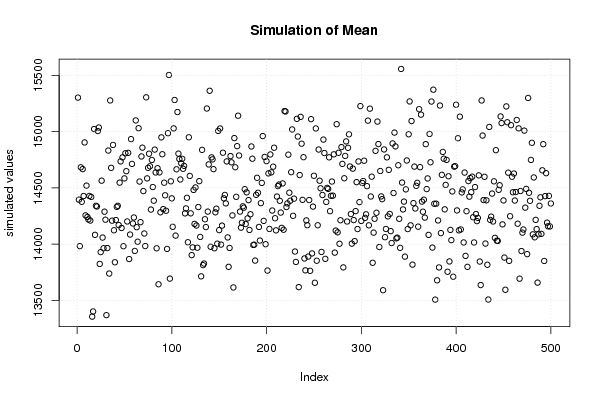PNG link Postscript link PDF link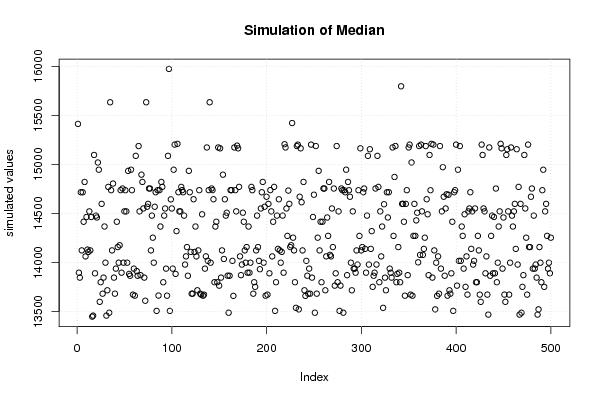PNG link Postscript link PDF link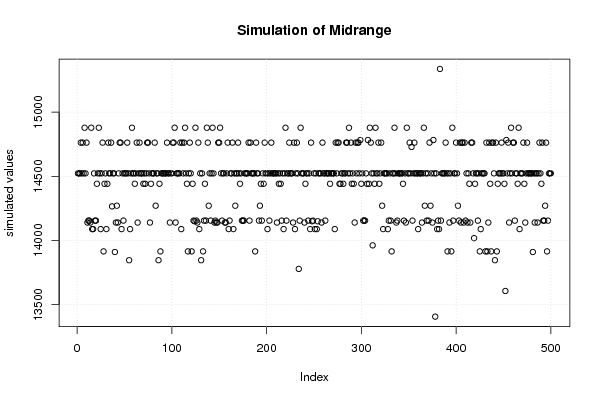PNG link Postscript link PDF link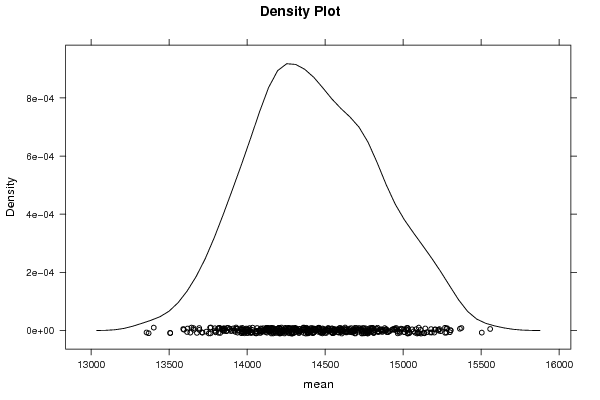PNG link Postscript link PDF link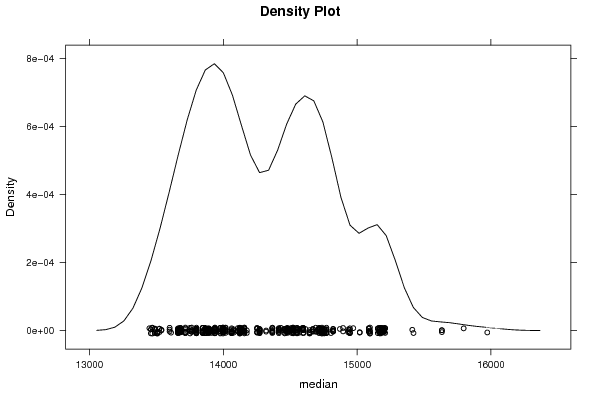PNG link Postscript link PDF link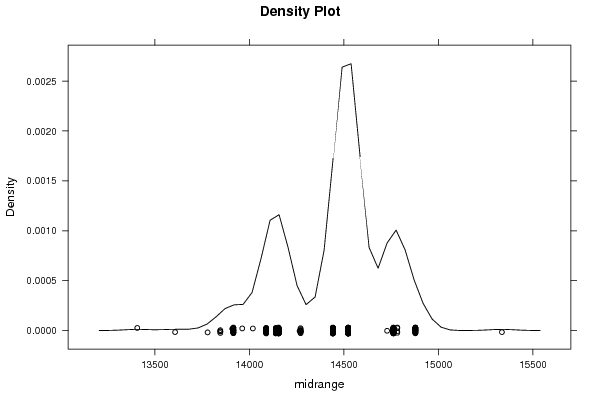PNG link Postscript link PDF link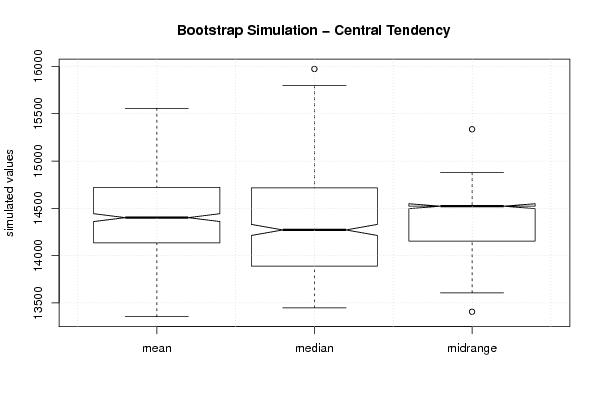PNG link Postscript link PDF link

Parameters (Session):
par1 = 500 ; par2 = 12 ;
Parameters (R input):
par1 = 500 ; par2 = 12 ;
R code (references can be found in the software module):
par1 <- as.numeric(par1)par2 <- as.numeric(par2)if (par1 < 10) par1 = 10if (par1 > 5000) par1 = 5000if (par2 < 3) par2 = 3if (par2 > length(x)) par2 = length(x)library(lattice)library(boot)boot.stat <- function(s){s.mean <- mean(s)s.median <- median(s)s.midrange <- (max(s) + min(s)) / 2c(s.mean, s.median, s.midrange)}(r <- tsboot(x, boot.stat, R=par1, l=12, sim='fixed'))bitmap(file='plot1.png')plot(r$t[,1],type='p',ylab='simulated values',main='Simulation of Mean')grid()dev.off()bitmap(file='plot2.png')plot(r$t[,2],type='p',ylab='simulated values',main='Simulation of Median')grid()dev.off()bitmap(file='plot3.png')plot(r$t[,3],type='p',ylab='simulated values',main='Simulation of Midrange')grid()dev.off()bitmap(file='plot4.png')densityplot(~r$t[,1],col='black',main='Density Plot',xlab='mean')dev.off()bitmap(file='plot5.png')densityplot(~r$t[,2],col='black',main='Density Plot',xlab='median')dev.off()bitmap(file='plot6.png')densityplot(~r$t[,3],col='black',main='Density Plot',xlab='midrange')dev.off()z <- data.frame(cbind(r$t[,1],r$t[,2],r$t[,3]))colnames(z) <- list('mean','median','midrange')bitmap(file='plot7.png')boxplot(z,notch=TRUE,ylab='simulated values',main='Bootstrap Simulation - Central Tendency')grid()dev.off()load(file='createtable')a<-table.start()a<-table.row.start(a)a<-table.element(a,'Estimation Results of Blocked Bootstrap',6,TRUE)a<-table.row.end(a)a<-table.row.start(a)a<-table.element(a,'statistic',header=TRUE)a<-table.element(a,'Q1',header=TRUE)a<-table.element(a,'Estimate',header=TRUE)a<-table.element(a,'Q3',header=TRUE)a<-table.element(a,'S.D.',header=TRUE)a<-table.element(a,'IQR',header=TRUE)a<-table.row.end(a)a<-table.row.start(a)a<-table.element(a,'mean',header=TRUE)q1 <- quantile(r$t[,1],0.25)[]q3 <- quantile(r$t[,1],0.75)[]a<-table.element(a,q1)a<-table.element(a,r$t0)a<-table.element(a,q3)a<-table.element(a,sqrt(var(r$t[,1])))a<-table.element(a,q3-q1)a<-table.row.end(a)a<-table.row.start(a)a<-table.element(a,'median',header=TRUE)q1 <- quantile(r$t[,2],0.25)[]q3 <- quantile(r$t[,2],0.75)[]a<-table.element(a,q1)a<-table.element(a,r$t0)a<-table.element(a,q3)a<-table.element(a,sqrt(var(r$t[,2])))a<-table.element(a,q3-q1)a<-table.row.end(a)a<-table.row.start(a)a<-table.element(a,'midrange',header=TRUE)q1 <- quantile(r$t[,3],0.25)[]q3 <- quantile(r$t[,3],0.75)[]a<-table.element(a,q1)a<-table.element(a,r$t0)a<-table.element(a,q3)a<-table.element(a,sqrt(var(r\$t[,3])))a<-table.element(a,q3-q1)a<-table.row.end(a)a<-table.end(a)table.save(a,file='mytable.tab')